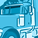# QlikView App Dev

Discussion Board for collaboration related to QlikView App Development.

Announcements
Support Case Portal has moved to Qlik Community! Read the FAQs to start exploring Support resources.
cancel
Showing results for
Did you mean:Partner

## Sum and count in same expression

Hello

Hope all is well with everyone.

We are trying to do sum and count of in the same expression but are not getting it right.

We have devices  (log_tun_id) and for each device we have number of packets (log_id_pk). What we want to do is for each device (log_tun_id) we would like to show how many times the packets (log_id_pk) is greater than certain value.

We are using the following expression but it is not working.  Seems like Sum and count does not work in the same expression?

Dimension: log_tun_id

Expression: Sum(if(count log_id_pk > 5, 1, 0) )

Will appreciate if someone can assist.

Thanks.

Message was edited by: Syed Jawwad Hussain

Message was edited by: Syed Jawwad Hussain Sorry actually the requirements was quoted wrong to me. The following are the correct requirements. * The count of log_id_pk for each device (tun_id_pk) should be equal to 15 * We want to find out the number of days when the count of log_id_pk for each tun_id_pk > 15 * Selecting the device should give us the list of days and the count where the count has been greater than 15 What i have tried is the following: dimension: log_tun_id expression: sum(aggr(if(count(log_id_pk)>3,1,0), datetimeindata_date, log_tun_id)) And it seems to be working fine. Thanks to all for the assistance as it really helped me to do the expression

1 Solution

Accepted SolutionsPartner

This expression

=Sum(Aggr(if(count(log_id_pk) > 5, 1, 0), tun_id_pk))

is syntactically correct for the information you have supplied. If that is not working, there is some other factor coming into play. If you upload a small sample qvw file together with the results you expect, then we can do more than just make wild guesses at the solution.

Logic will get you from a to b. Imagination will take you everywhere. - A Einstein
16 RepliesSpecialist II

try like

SUM(aggr(count(if(log_id_pk > 5,log_tun_id)),log_tun_id))MVP

You need to use advanced aggregation if you want to embed a count in a another aggregation function.

But maybe this is sufficient?

=Sum( log_id_pk >5 ) *-1

Sum( Aggr( Sum(If( Log_id_pk >5,1)), log_tun_id))Partner
Author

Tim,

Thanks for the quick response.  Our QV server is down at the moment and i will try as soon as it is up.

Why do we have to use the aggregate function? it could not work with simple sum(if(count condition),1,0)?

Also can you briefly explain the aggr function as i get confused whether to use it inside the count or outside.

Thanks.Partner
Author

Dear Stefan,

Thanks for your quick response. Our server is down at the moment and i will enter your expression as soon as it is up.

=Sum( log_id_pk >5 ) *-1

- Actually we have Raw Transaction Table so we cannot aggregate the counts as we have to use them for other expressions.

- What is the purpose of *-1? after summation?

Thanks.MVP
 =Sum( log_id_pk >5 ) *-1 - Actually we have Raw Transaction Table so we cannot aggregate the counts as we have to use them for other expressions. - What is the purpose of *-1? after summation?

Could you elaborate on your first statement? How does your data model look like? Could you post some sample records?

Referring to the second question: when executing  log_id_pk >5 on row level, a boolean value is returned TRUE or FALSE, or in numeric representation -1 or 0. Summing up the TRUE values returns a negative value, hence you would need to multiply with -1 (or use fabs() ) to get your positive value equal to a count of rows where log_id_pk >5Specialist II

you cant use two aggregation functions like Count and sum in one Expression.

There is were the aggr() Comes into Play. the aggr function created a temporary table of Content.

in this example aggr(count(if(log_id_pk > 5,log_tun_id)),log_tun_id) creates a table like this

Log_tun_id          Count(if(log_id_pk>5, log_tun_id))

1                          12

2                          14

3                          16

4                          17

Now we want to get the sum of this, so we surround the Expression above with sum().

-> sum(  aggr(count(if(log_id_pk > 5,log_tun_id))  )

hope this helpsSpecialist III

Hi Syed:

Sum({<log_id_pk = {"=Count(log_id_pk)>5"}>} 1)

Regards!MVP

Hi,

Try like this

Sum(Aggr(if(count(log_id_pk) > 5, 1, 0),  log_id_pk))

OR

Sum({<log_id_pk = {"=Count(log_id_pk)>5"}>} DISITNCT log_id_pk)

Hope this helps you.

Regars,

Jagan.Partner
Author

I tried your suggestion but it is not working.  It is giving all zeros.

I tried to switch the count and the if - sum(  aggr(if(count(log_id_pk > 5),log_tun_id))  )

But it is not working properly.Community Browser